# Question 4

• Potassium Chromate is a yellowish, crystalline compound.# Question 10

• First Ionization Energy: He > Ne > Ar > Kr > Xe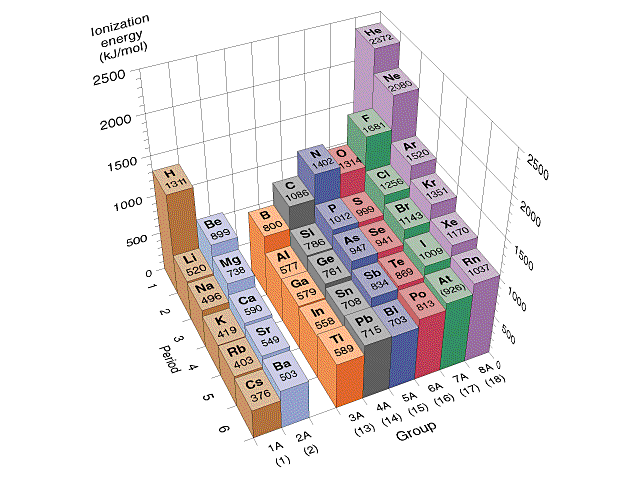# Question 15

• A typical Lewis acid-base reaction: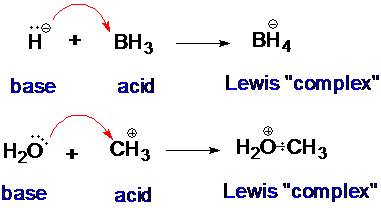# Question 23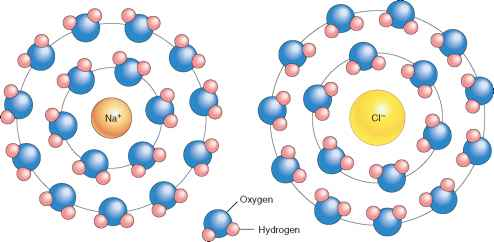• For the process of solid calcium chloride dissolving in water, the entropy change is negative since water molecules in the hydration shells of Ca2+ and Cl- ions are more ordered than they are in the pure water

# Question 28# Question 30# Question 34

• N2H4 exhibits significant hydrogen bonding in the liquid state.# Question 37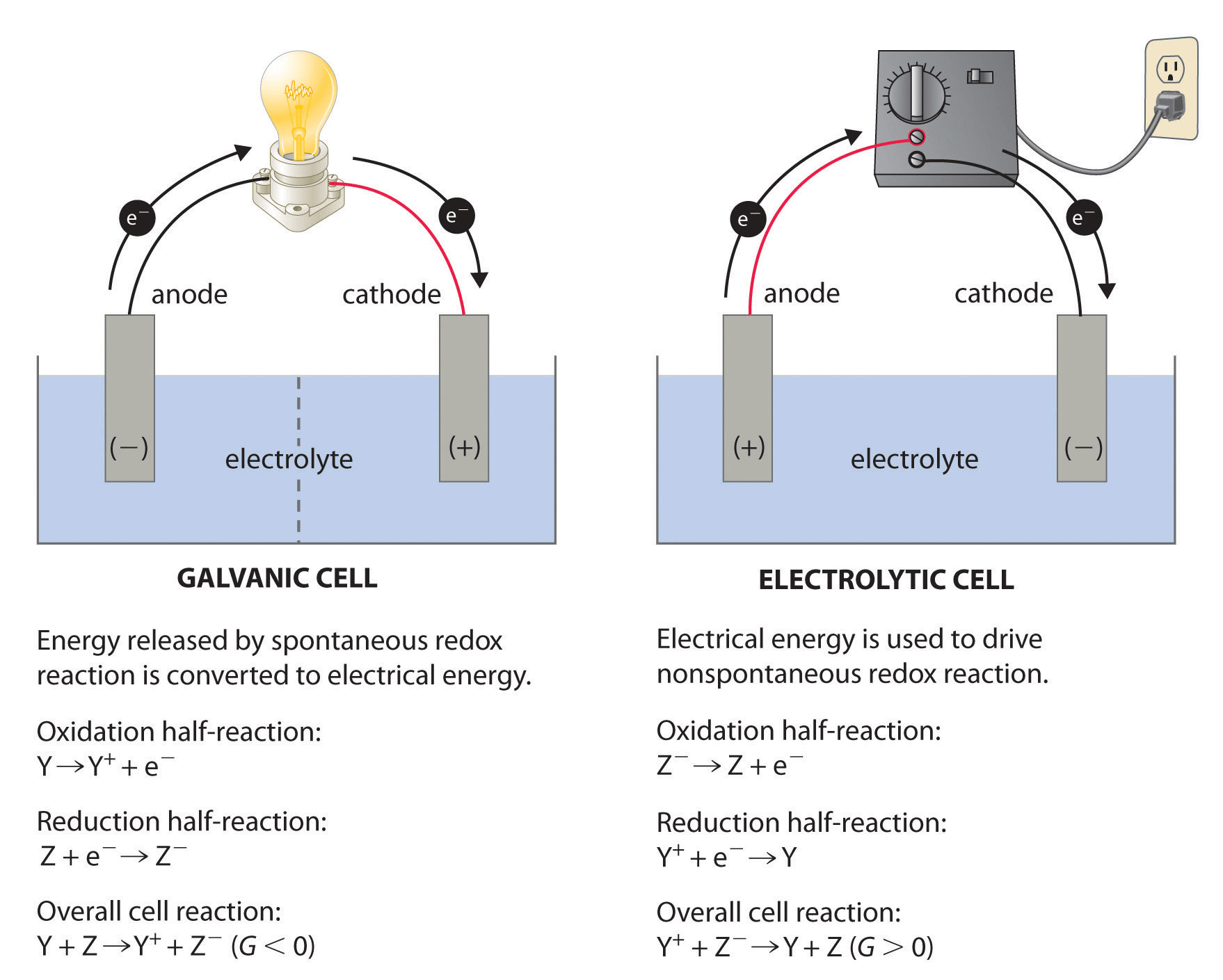# Question 38

• Calculate standard cell potential for the galvanic cell:# Question 40

• Beta plus decay vs Beta minus decay# Question 47

![Zeroth Order First Order Second Order Differential rate law Concentration vs. time Integrated rate law Straight-line plot to determine rate constant Relative rate vs. concentration Half-life Units of k, rate constant Rate = o o o o ALA] At Time Slope = —k Time Rate — o o ALA] = MA] At Time o o —kt or Slope = —k Time [A], M Rate, M/S o o o o 2 3 M Rate, M/s 2k 2 3 Rate = 2 3 A[A] = At Time Slope = k Time Rate, M/S 4 9 2 3 0.693 l/s ](./media/image22.png)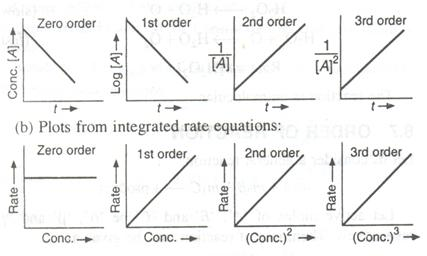# Question 51

• O3 contains bonds that have a bond order of 1.5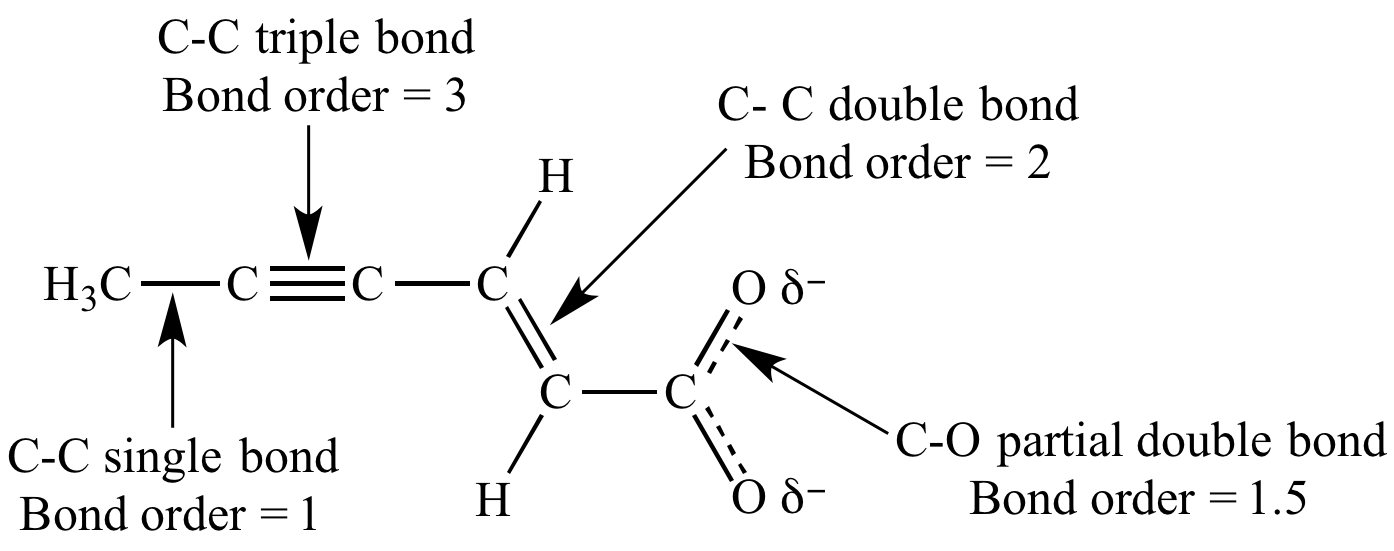# Question 67# Question 71

• Endothermic: Separation of solute and solvent

• Exothermic: Intermolecular attractions form between solute and solvent